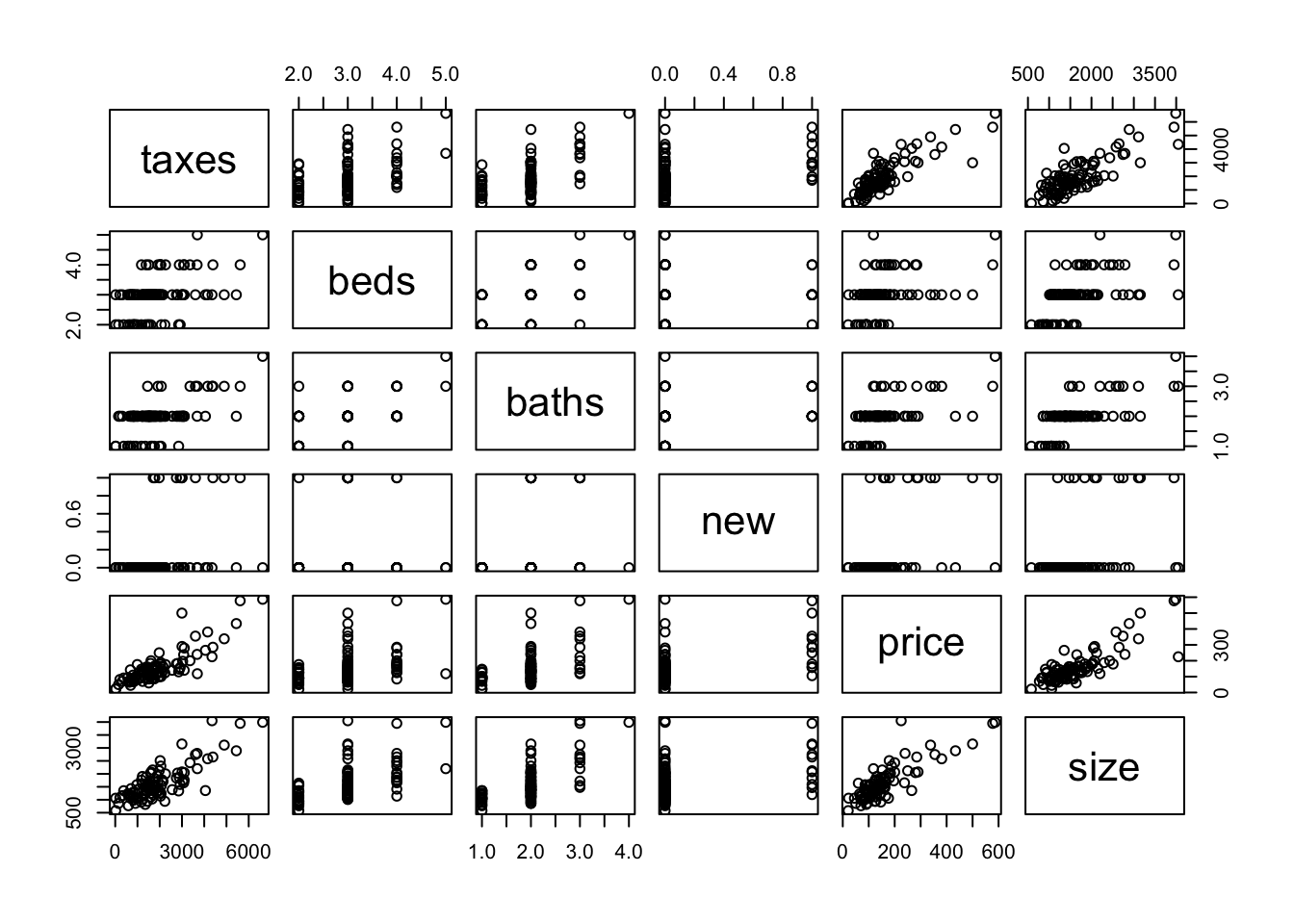# 1. Data

We use the same dataset as Agresti Chapter 4.7. The question here is to understand the questioni: what affects the selling price of a house? This dataset contains $$100$$ house selling records in Gainesville, Florida.

Houses <- read.table("Houses.dat", header = T)
Houses
• price: selling price of the house in thousands of dollars.
• size: size of the house in square feet
• taxes: annual property tax
• beds: number of bedrooms
• baths: number of bathrooms
• new: whether the house is a new construction or not

## 1.1 Some data exploration

Pair-wise scattor plots

pairs(Houses[, -1])Pair-wise correlations

cor(Houses[, -1])
##           taxes       beds     baths        new     price      size
## taxes 1.0000000 0.47392873 0.5948543 0.38087410 0.8419802 0.8187958
## beds  0.4739287 1.00000000 0.4922224 0.04931556 0.3939570 0.5447831
## baths 0.5948543 0.49222235 1.0000000 0.25148095 0.5582533 0.6582247
## new   0.3808741 0.04931556 0.2514810 1.00000000 0.4732608 0.3843277
## price 0.8419802 0.39395702 0.5582533 0.47326080 1.0000000 0.8337848
## size  0.8187958 0.54478311 0.6582247 0.38432773 0.8337848 1.0000000

# 2. Using a linear model

We include all covariates, and can decide whether we also want to include interactions

We can compare the model with and without interactions.

fit.1 <- lm(price ~ size + new + baths + beds + taxes, data = Houses)
fit.2 <- lm(price ~ (size + new + beds + baths + taxes)^2, data = Houses)
anova(fit.1, fit.2, test = "Chisq")

If we use the glm function, the default is also to use the Gaussian linear model, though the ANOVA table column names are different.

fit.1 <- glm(price ~ size + new + baths + beds + taxes, data = Houses)
fit.2 <- glm(price ~ (size + new + baths + beds + taxes)^2, data = Houses)
anova(fit.1, fit.2, test = "Chisq")

One thing to notice is that the deviance and residual deviance reported here are values with $$\sigma^2 = 1$$. The computation of p-values takes into account the estimation of $$\sigma^2$$.

We can even compare with a model adding third order interactions.

fit.3 <- glm(price ~ (size + new + beds + baths + taxes)^3, data = Houses)
anova(fit.2, fit.3, test = "Chisq")

We see that the third-order interactions also seems help.

## 2.1 Model checking for the linear models

All the above p-values are valid only when the equal variance assumption holds. So before we further select a good linear model, we should check whether the residuals look good.

par(mfrow=c(1,3), mar = c(4, 4, 6, 4))
plot(fit.1, which = 3, main = "main effect model")
plot(fit.2, which = 3, main = "second-order interations")
plot(fit.3, which = 3, main = "third-order interactions")
## Warning: not plotting observations with leverage one:
##   22, 35, 68, 84, 92